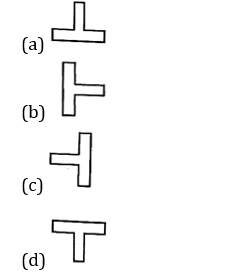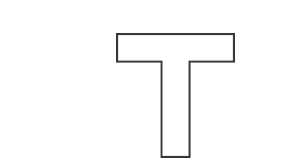Engineering Jobs   »   CIVIL ENGINEERING QUIZ

# DFCCIL’21 CE: Daily Practice Quiz. 30-July-2021

Quiz: Civil Engineering
Exam: DFCCIL-JE
Topic: MISCELLANEOUS

Each question carries 1 mark
Negative marking: 1/4 mark
Time: 8 Minutes

Q1. For a given elastic material, the elastic modules E is 210 GPa and its Poisson’s Ratio is 0.27. What is the approximate value of its modules of rigidity?
(a) 105 GPa
(b) 83 GPa
(c) 159 GPa
(d) 165 GPa

Q2. A simply supported beam of span ‘L’ is loaded with downward uniformly distributed load of intensity W/m over its entire length. Which of the following orientation of T beams is preferred to resist bending?Q3. If all the dimensions of a prismatic bar of square cross-section suspended freely from the ceiling of a roof are doubled then the total elongation produced by its own weight will increase.
(a) Eight times
(b) Four times
(c) Three times
(d) Two times

Q4. Angle of twist of a circular shaft under the action of a torsional moment T is given by
(a) GJ/TL
(b) TL/GJ
(c) TJ/GL
(d) TG/JL

Q5. Which of the following materials has the highest carbon percentage?
(a) Cast iron
(b) Mild steel
(c) High carbon steel
(d) Stainless steel

Q6. The plasticity to mould bricks in suitable shape is contributed by
(a) Alumina
(b) Lime
(c) Magnesia
(d) Silica

SOLUTION

S1. Ans.(b)
Sol. Given, Elastic modulus (E) = 210 GPa
Poison ratio (μ) = 0.27
Modulus of rigidity (G) = ?
E = 2G(1+μ)
G =E/2(1+μ)
=210/2(1+0.27)
▭(G=82.67 GPa≈83 GPa)

S2. Ans.(d)
Sol.(it is the best orientation of T-beam to resist the bending)

S3. Ans.(b)
Sol. Total elongation in a prismatic bar due to its self-weight is given by-
▭(∆L=(yL^2)/2E)
Now, length of bar is doubles then Total elongation in bar will be-
∆L^’=(γ×(2L)^2)/2E
=4×(γL^2)/2E
▭(∆L^’=4∆L)

S4. Ans.(b)
Sol. form the Torsion equation →
T/J=Gθ/L
▭(θ=TL/GJ)
Where θ = angle of twist
T= Applied twisting moment
L= Length of the shaft
G= Modulus of rigidity
J= Polar moment of inertia

S5. Ans.(a)
Sol.
Type of steel Carbon percentage
Cast iron 2.0 – 4.0%
Steel 0.1 – 0.25%
Wrought iron (purest form of iron) < 0.1 %
If carbon content (%) is higher, then the material will be more brittle and lesser will lead to ductile material.

S6. Ans.(a)
Sol. Alumina imparts plasticity to bricks. If it is in excess then shrinkage and warping of bricks takes place. The percentage of alumina in good brick earth is 20-30 %.

Sharing is caring!